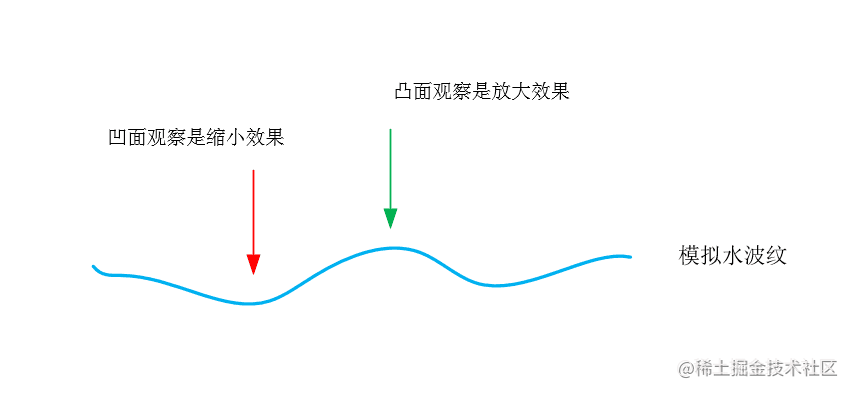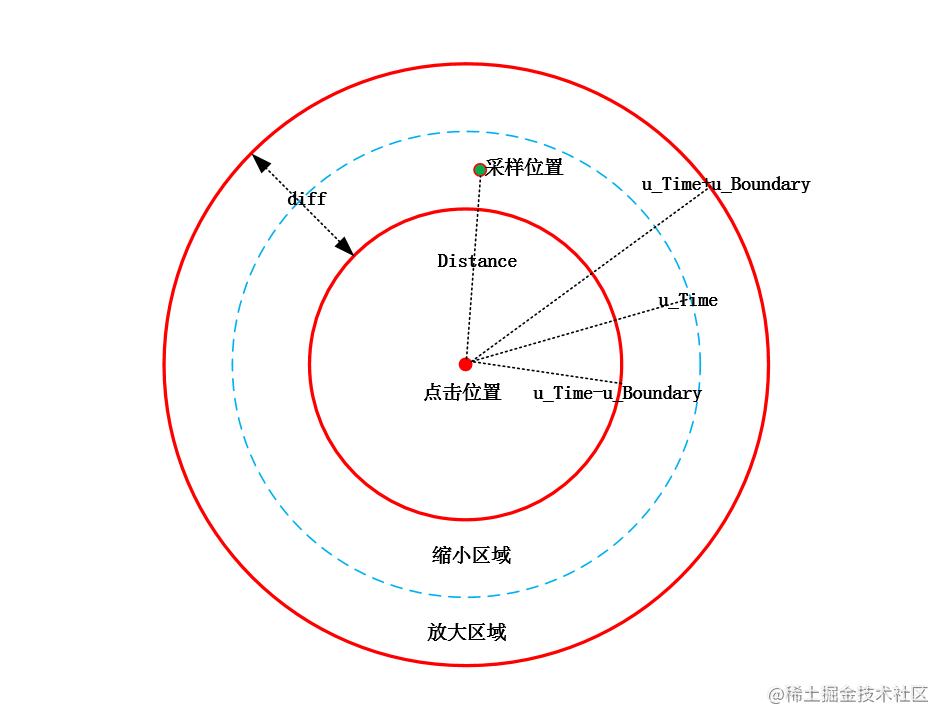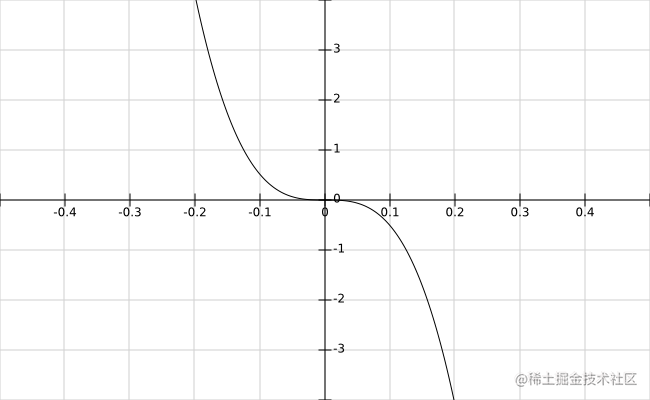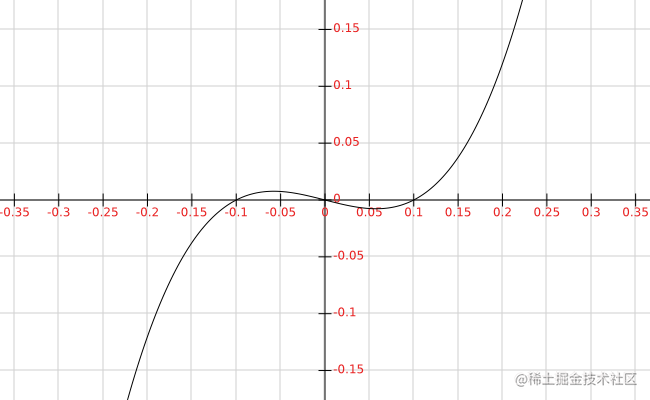# Android OpenGL ES 实现动态（水波纹）涟漪效果# 水波纹效果原理# 水波纹效果实现``````#version 300 es
precision highp float;
in vec2 v_texCoord;
layout(location = 0) out vec4 outColor;
uniform sampler2D s_TextureMap;//采样器
uniform vec2 u_TouchXY;//点击的位置（归一化）
uniform vec2 u_TexSize;//纹理尺寸
uniform float u_Time;//归一化的时间
uniform float u_Boundary;//边界 0.1
void main()
{
float ratio = u_TexSize.y / u_TexSize.x;
vec2 texCoord = v_texCoord * vec2(1.0, ratio);//根据纹理尺寸，对采用坐标进行转换
vec2 touchXY = u_TouchXY * vec2(1.0, ratio);//根据纹理尺寸，对中心点坐标进行转换
float distance = distance(texCoord, touchXY);//采样点坐标与中心点的距离

if ((u_Time - u_Boundary) > 0.0
&& (distance <= (u_Time + u_Boundary))
&& (distance >= (u_Time - u_Boundary))) {
float diff = (distance - u_Time); //输入 diff
float moveDis =  - pow(8 * diff, 3.0);//平滑函数 -(8x)^3 采样坐标移动距离
vec2 unitDirectionVec = normalize(texCoord - touchXY);//单位方向向量
texCoord = texCoord + (unitDirectionVec * moveDis);//采样坐标偏移（实现放大和缩小效果）
}

texCoord = texCoord / vec2(1.0, ratio);//转换回来
outColor = texture(s_TextureMap, texCoord);
}

``````void ShockWaveSample::Draw(int screenW, int screenH)
{
LOGCATE("ShockWaveSample::Draw()");
m_SurfaceWidth = screenW;
m_SurfaceHeight = screenH;
if(m_ProgramObj == GL_NONE || m_TextureId == GL_NONE) return;

m_FrameIndex ++;

UpdateMVPMatrix(m_MVPMatrix, m_AngleX, m_AngleY, (float)screenW / screenH);

glUseProgram (m_ProgramObj);
glBindVertexArray(m_VaoId);

GLUtils::setMat4(m_ProgramObj, "u_MVPMatrix", m_MVPMatrix);

glActiveTexture(GL_TEXTURE0);
glBindTexture(GL_TEXTURE_2D, m_TextureId);
GLUtils::setFloat(m_ProgramObj, "s_TextureMap", 0);

//float time = static_cast<float>(fmod(GetSysCurrentTime(), 2000) / 2000);
float time = static_cast<float>(fmod(m_FrameIndex, 150) / 120);
GLUtils::setFloat(m_ProgramObj, "u_Time", time);

//设置点击位置
GLUtils::setVec2(m_ProgramObj, "u_TouchXY", m_touchXY);
//设置纹理尺寸
GLUtils::setVec2(m_ProgramObj, "u_TexSize", vec2(m_RenderImage.width, m_RenderImage.height));
//设置边界值
GLUtils::setFloat(m_ProgramObj, "u_Boundary", 0.1f);

glDrawElements(GL_TRIANGLES, 6, GL_UNSIGNED_SHORT, (const void *)0);

}``````float x = (distance - u_Time); //输入 diff = x
float moveDis = 20.0 * x * (x - 0.1)*(x + 0.1);//平滑函数 y=20.0 * x * (x - 0.1)*(x + 0.1)  采样坐标移动距离Android